# Concepts of Thermodynamics

L#3

## ZEROTH LAW OF THERMODYNAMICS

If objects A and B are separately in thermal equilibrium with a third object C then objects A and B are in thermal equilibrium with each other. Zeroth law of thermodynamics introduces thermodynamic quantity called temperature. Two objects (or systems) are said to be in thermal equilibrium if their temperatures are the same. In measuring the temperature of a body, it is important that the thermometer be in the thermal equilibrium with the body whose temperature is to be measured.

## FIRST LAW OF THERMODYNAMICS

The first law of thermodynamics is based on conservation of energy. According to this law heat Q supplied to a system is equal to the sum of the change in internal energy (DU) and work done by the system (W). Thus we can write

Q = DU + W

### More about First Law of Thermodynamics

1. Heat supplied to the system taken as positive and heat given by the system taken as negative.

2. It makes no different between heat and work. It does not indicate that why the whole of heat energy cannot be converted into work.

3. Heat and work depend on the initial and final states but on the path also. The change in internal energy depends only on initial and final states of the system.

4. The work done by the system against constant pressure P is W = PDV.

So the first law of thermodynamics can be written as:

Q = D +D U PV .

5. Differential form of the first law;

dQ = dU + dW

or              dQ = dU + PdV

## ENTROPY

Entropy is the another thermodynamical variable which many times very useful to understand the system. Entropy is related to the disorder or randomness in the system. To understand this, let us consider two systems as shown in Fig.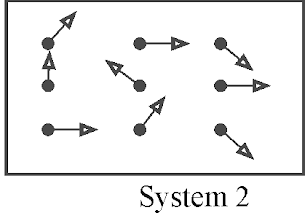If S1
and S2 are the entropies of the system 1 and 2 respectively at any temperature, then S1< S2.

(i) Entropy is not a conserved quantity.

(iii) Entropy of the universe always increases.If a system at temperature T is supplied a small amount of heat DQ, then change in entropy of the system can be defined as

DS = DQ / T for constant T

For a system with variable T, we have The second law of thermodynamics may be stated in terms of entropy as:

It is impossible to have a process in which the entropy of an isolated system is decreased

## THERMODYNAMICAL PROCESSES

Any process may have own equation of state, but each thermodynamical process must obey PV = nRT.

1. Isobaric Process :

If a termodyanomic system undergoes physical change at constant pressure,then the proccess is called isoparic.

(i) Isobaric process obeys Charle’s law, VαT

(ii) Slope of P~ V curve DP / DV=0

(iii) Specific heat at constant pressure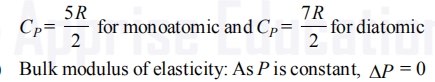(iv) Bulk modulus of elasticity: As P is constant, DP = 0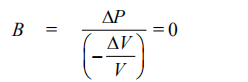(v)Work done :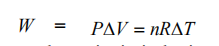(vi) First law of thermodynamics in isobaric process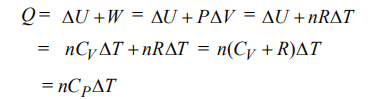## 2. Isochoric or Isometric Process :

A thermodynamical process in which volume of the system remain constant, is called isochoric process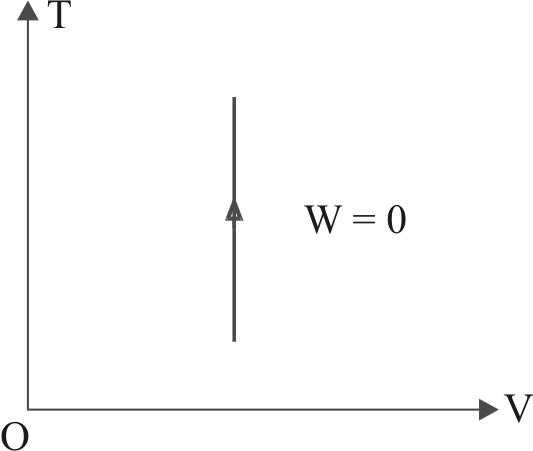(i) An isochoric process obeys Gay - Lussac’s Law, P µ T

(ii) Slope of P – V curve,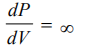(iii) Specific heat at constant volume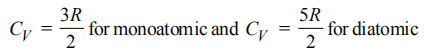(vi) Bulk modulus of elasticity: As V is constant, DV=0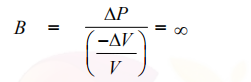(v) Work done :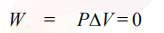(vi) First law of thermodynamics in isochoric process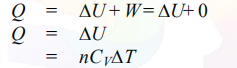3.Isothermal process:-

A thermodynomical process in which pressure and volume of the system change at constant temperature, is called isothemal process.

(i) An isothermal process obeys Boyle’s law PV = constant

(ii) The wall of the container must be perfectlyconducting so that free exchange of heat between the system and surroundings can take place..

(iii) The process must be very slow, so as to provide sufficient time for the exchange of heat.

(iv) Slope of P – V curve:

For isothermal process

PV = Constant

After differentiating w.r.t. volume, we get(v) Specific heat at constant temperature: As DT = 0,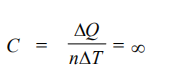(vi) First law of thermodynamics in isothermal process.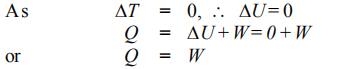An adiabatic process is one in which pressure, volume and temperature of the system change but heat will not exchange between system and surroundings.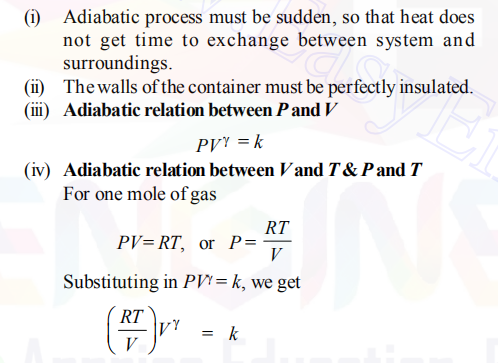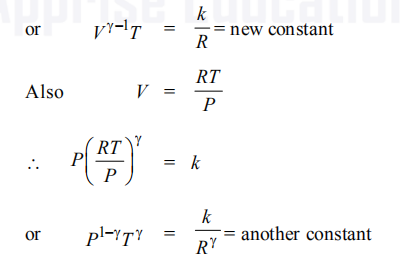Specific heat: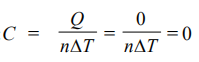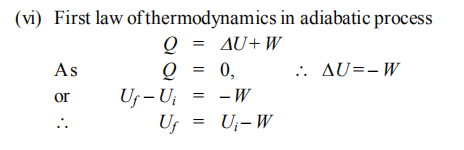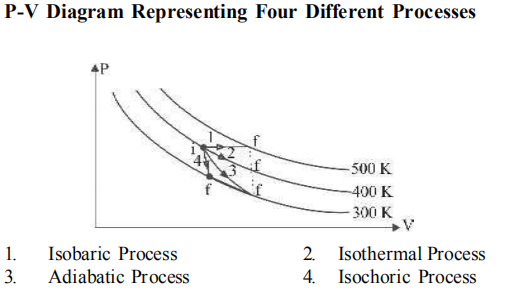thank you visit and share..